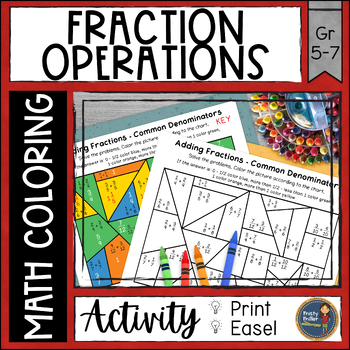# Fraction Operations Math Color Pages

Rated 4.96 out of 5, based on 110 reviews
110 Ratings;
6th - 7th, Homeschool
Subjects
Resource Type
Standards
Formats Included
• PDF
Pages
12 pages
\$3.60
List Price:
\$4.50
You Save:
\$0.90
\$3.60
List Price:
\$4.50
You Save:
\$0.90
Report this resource to TPT
Easel Activity Included
This resource includes a ready-to-use interactive activity students can complete on any device.  Easel by TPT is free to use! Learn more.

### Description

Practice fraction operations with these math coloring pages. Students solve problems by adding, subtracting, multiplying, and dividing fractions. Then they use the code to color the picture. Fraction Operations Color with Math includes 6 problem/color sheets and answer keys. Students will need to simplify some problems and change improper fractions to mixed numbers.

What's Included:

→ Adding Fractions with Common Denominators (1 sheet)

→ Adding Fractions with Uncommon Denominators (1 sheet)

→ Subtracting Fractions with Common Denominators (1 sheet)

→ Subtracting Fractions with Uncommon Denominators (1 sheet)

→ Multiplying Fractions (1 sheet)

→ Dividing Fractions (1 sheet)

This resource is great for:

✧ Distance learning

✧ Independent work

✧ Math Centers, Math Stations

✧ At Home Learning, Homework

✧ Skill Practice or Review

Similar Resources & Skills:

◈ ◈ ◈ ◈ ◈ ◈ ◈ ◈ ◈ ◈ ◈ ◈ ◈ ◈ ◈ ◈ ◈ ◈ ◈ ◈ ◈ ◈ ◈ ◈ ◈ ◈ ◈ ◈ ◈ ◈ ◈ ◈ ◈ ◈ ◈ ◈ ◈ ◈

➯ Don't forget to leave feedback. You will receive TPT credits that can be used on future purchases!

Total Pages
12 pages
Included
Teaching Duration
Other
Report this resource to TPT
Reported resources will be reviewed by our team. Report this resource to let us know if this resource violates TPT’s content guidelines.

### Standards

to see state-specific standards (only available in the US).
Add and subtract fractions with unlike denominators (including mixed numbers) by replacing given fractions with equivalent fractions in such a way as to produce an equivalent sum or difference of fractions with like denominators. For example, 2/3 + 5/4 = 8/12 + 15/12 = 23/12. (In general, 𝘢/𝘣 + 𝘤/𝘥 = (𝘢𝘥 + 𝘣𝘤)/𝘣𝘥.)
Apply and extend previous understandings of multiplication to multiply a fraction or whole number by a fraction.
Interpret and compute quotients of fractions, and solve word problems involving division of fractions by fractions, e.g., by using visual fraction models and equations to represent the problem. For example, create a story context for (2/3) ÷ (3/4) and use a visual fraction model to show the quotient; use the relationship between multiplication and division to explain that (2/3) ÷ (3/4) = 8/9 because 3/4 of 8/9 is 2/3. (In general, (𝘢/𝘣) ÷ (𝘤/𝘥) = 𝘢𝘥/𝘣𝘤.) How much chocolate will each person get if 3 people share 1/2 lb of chocolate equally? How many 3/4-cup servings are in 2/3 of a cup of yogurt? How wide is a rectangular strip of land with length 3/4 mi and area 1/2 square mi?